Maths-
General
Easy

Question

# If the length of the median of an equilateral triangle is x cm, then what is the area of triangle.Hint:

## The correct answer is: Equilateral triangle

### It is given that length of the median = x cmLet side of the triangle = a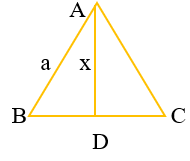Since median divides the base ⇒ BD =Using Pythagoras in triangle ABDAB2 = AD2 + BD2a2 = x2 + (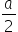)2= x2  ⇒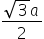= xa =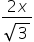Area of equilateral triangle =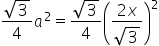=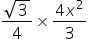=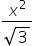#### With Turito Foundation.#### Get an Expert Advice From Turito.